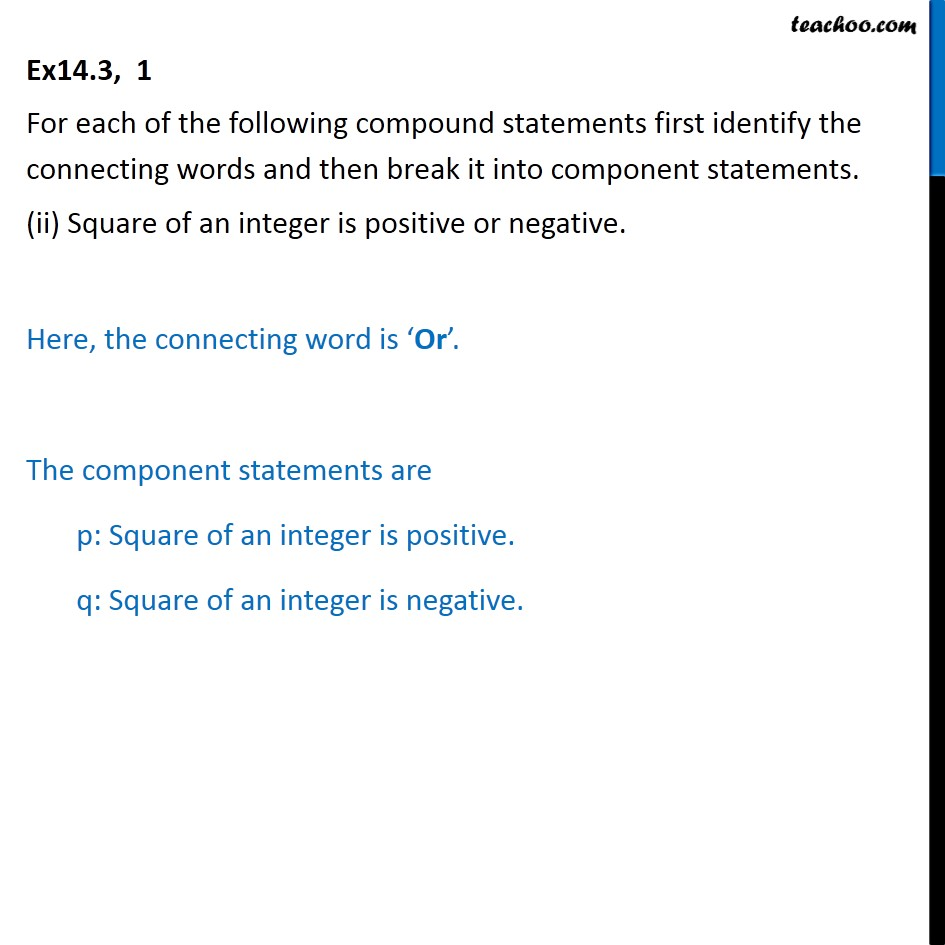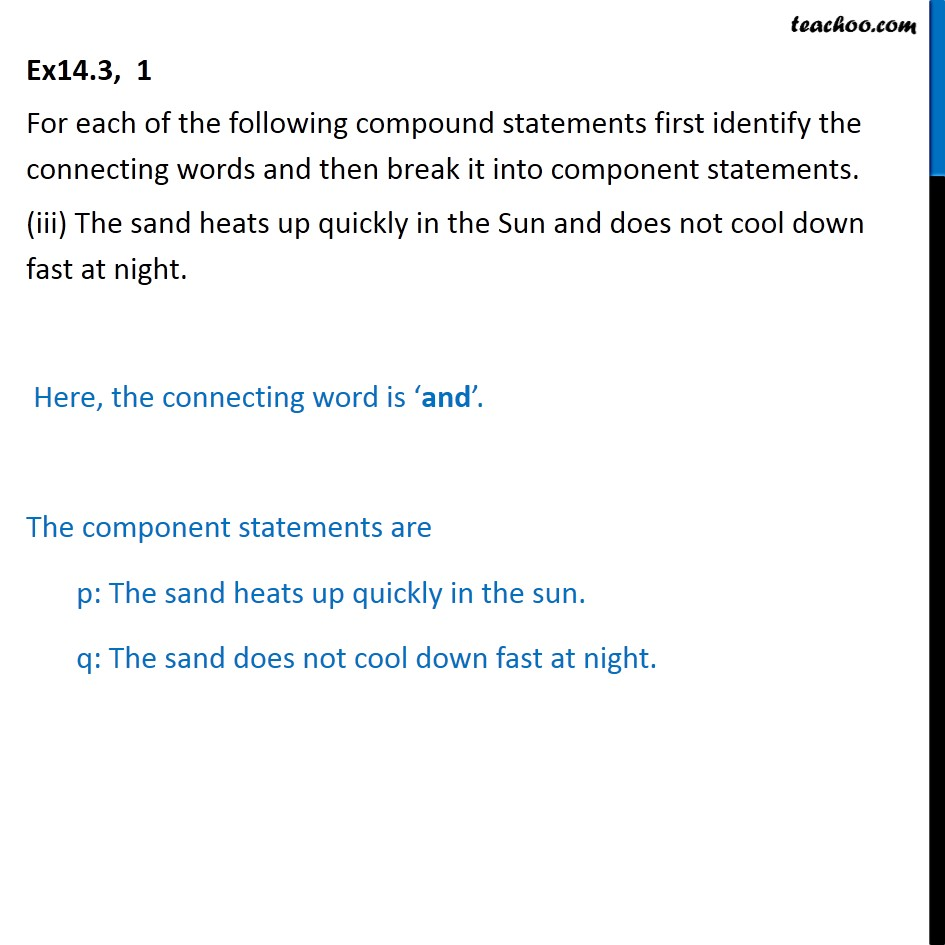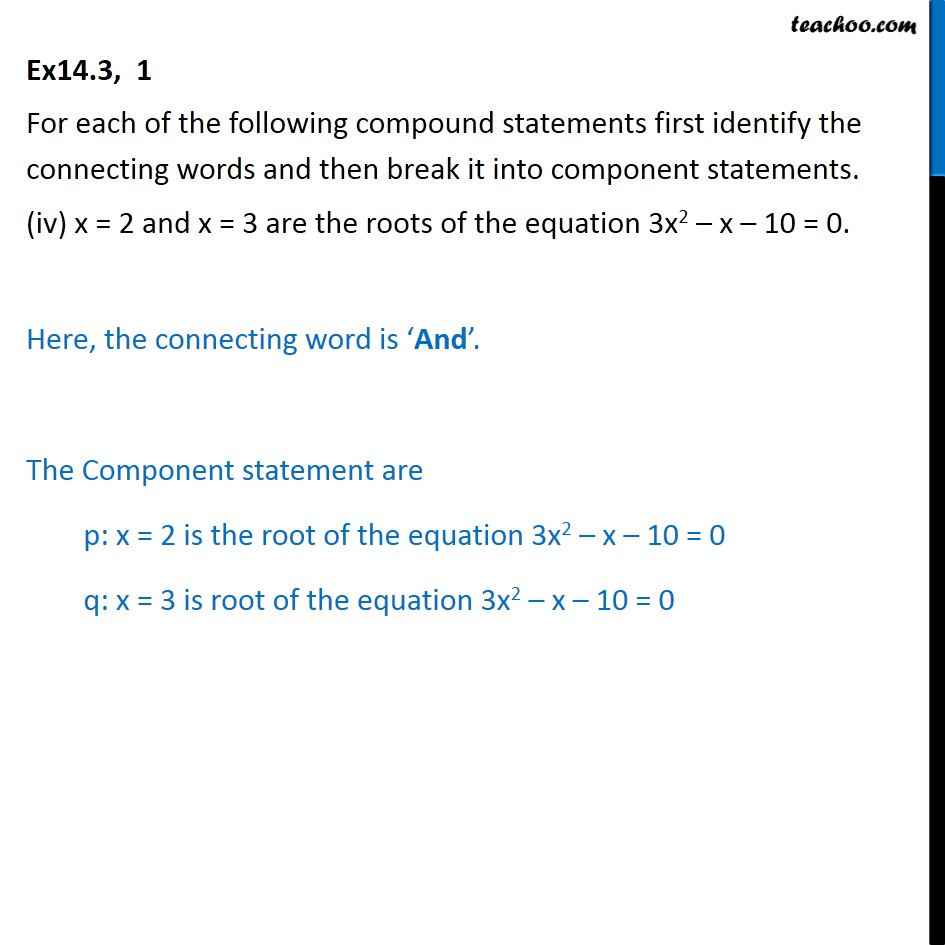Subscribe to our Youtube Channel - https://you.tube/teachoo

1. Chapter 14 Class 11 Mathematical Reasoning
2. Serial order wise
3. Ex 14.3

Transcript

Ex 14.3, 1 For each of the following compound statements first identify the connecting words and then break it into component statements. (i) All rational numbers are real and all real numbers are not complex. Here, the connecting word is ‘and’. The component statements are p: All rational numbers are real. q: All real numbers are not complex. Ex14.3, 1 For each of the following compound statements first identify the connecting words and then break it into component statements. (ii) Square of an integer is positive or negative. Here, the connecting word is ‘Or’. The component statements are p: Square of an integer is positive. q: Square of an integer is negative. Ex14.3, 1 For each of the following compound statements first identify the connecting words and then break it into component statements. (iii) The sand heats up quickly in the Sun and does not cool down fast at night. Here, the connecting word is ‘and’. The component statements are p: The sand heats up quickly in the sun. q: The sand does not cool down fast at night. Ex14.3, 1 For each of the following compound statements first identify the connecting words and then break it into component statements. (iv) x = 2 and x = 3 are the roots of the equation 3x2 – x – 10 = 0. Here, the connecting word is ‘And’. The Component statement are p: x = 2 is the root of the equation 3x2 – x – 10 = 0 q: x = 3 is root of the equation 3x2 – x – 10 = 0

Ex 14.3